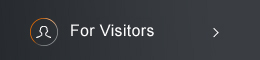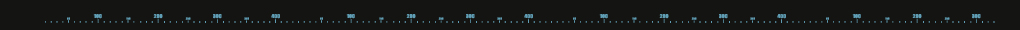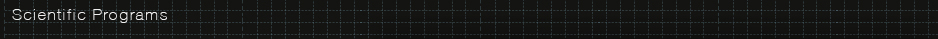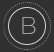Sponsored byAlgebraic Geometry
 Program Committee: Ching-Jui Lai (NCKU, committee chair), Hsueh-Yung Lin (NTU), Jeng-Daw Yu (NTU)   Description of program: Algebraic geometry studies the geometry of algebraic and analytic objects and it has been a core area of the modern mathematics for more than a century. Today it remains a very active area in mathematics and related fields with many of its techniques, notions, and methods having important applications in number theory, differential geometry, physics, and even in statistics and data analysis. Research areas of the Algebraic Geometry group at the NCTS include birational geometry, generalized Hodge theory, linear systems and positivity, instantons and quiver varieties, analytical methods and algebraic and complex geometry, topology and geometry of moduli spaces, and nonabelian cohomology. More detailed description of each topic is given below.   Affiliated members: Jungkai Chen (NTU), Shin-Yao Jow (NTHU), Ching-Jui Lai (NCKU), Hsueh-Yung Lin (NTU), Frank Liou (NCKU), Chih-Chung Liu (NCKU), Jeng-Daw Yu (NTU), Zhu Eugene Xia (NCKU)   Birational Geometry (Jung-Kai Chen, Jheng-Jie Chen, Ching-Jui Lai) The minimal model program (MMP) attempts to find canonical representatives for the birational equivalence class of varieties. The foundation of MMP in characteristic zero is outlined by Reid, Shokurov, Kawamata, Kollar, Mori, and further advanced by Birkar-Cascini-Hacon-McKernan and many others. There are also generalizations of MMP in positive characteristics, by using the Frobenius morphism and the Serre vanishing theorem. Our research program follows and aims to extend these work. For example, a detailed study of three dimensional elementary birational maps in MMP, which is expected to be well understood explicitly, can be generally useful in the study of Sarkisov program, rationality problem and Fano varieties, etc.   Generalized Hodge Theory and Applications (Jeng-Daw Yu) We focus on the developments of various generalizations of Hodge theory, in particular, the irregular Hodge theory, motivated by research in mirror symmetry, arithmetic, singularity theory, etc. Conversely, we explore the applications of the generalized Hodge theory to other research areas. Linear Systems and Positivity (Shin-Yao Jow) One way to study the geometry of an algebraic variety is to look at the families of subvarieties it contains. Linear systems are families of codimension-one subvarieties that vary linearly. They have been a useful tool in algebraic geometry since at least the time of the Italian school. In more modern language, a linear system is a linear subspace of sections of a line bundle, and the behavior of the linear system is intimately related to the positivity of the line bundle. There has been ongoing research on this theme: an example of recent development is the theory of Okounkov bodies, which brings in the perspective of convex geometry. Our research program actively follows the recent works on this theme and explores their various implications as well as their generalization to vector bundles of higher ranks. Instantons and Quiver Varieties (Chih-Chung Liu)  Quiver varieties have been used to describe instantons of Kähler-Einstein metrics via the constructions of asymptotically locally Euclidean (ALE) hyperkahler four manifolds. Our research program is active in studying other gauge theoretic equations from these algebraic aspects.   Dynamics in Algebraic Geometry (Hsueh-Yung Lin) We study self-maps and group actions in the context of algebraic geometry. Typical objects of study include biregular and birational automorphisms of a variety, as well as the groups they generate. We also study the induced actions on various invariants, e.g. the cohomology algebra and relevant positive cones therein. Dynamics in algebraic geometry contains a broad spectrum of topics ranging from Cremona groups to endofunctors of categories of geometric nature, some of which are naturally related to group theory, (classical or p-adic) analysis, and dynamical systems.   Topology and Geometry of Moduli Spaces (Jia-Ming Frank Liou) A moduli space is a geometric space whose points represent isomorphism classes of objects. Such a space arises from classification problems. For example, the moduli space of compact Riemann surfaces is an orbifold whose points are isomorphism classes of compact Riemann surfaces, the Hurwitz space is a complex manifold which classifies meromorphic functions on compact Riemann surfaces, and Grassmannians are complex manifolds which classify subspaces of a given vector space. Our main goal is to use complex analytic tools to study the geometry and topology of moduli spaces.   Moduli Spaces of Sheaves and Higgs Bundles (Zhu Eugene Xia) The algebraic geometry group is actively working on moduli spaces of sheaves and Higgs bundles; character varieties, mapping class groups,  and other related objects over curves and higher dimension varieties.(C) 2021 National Center for Theoretical Sciences